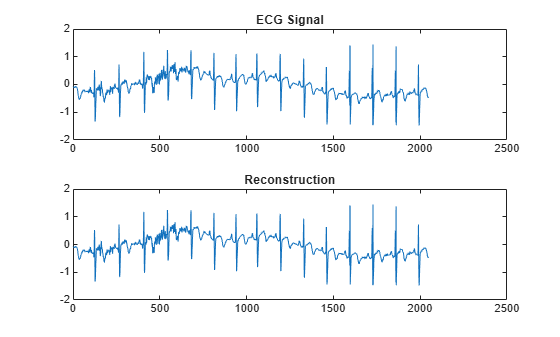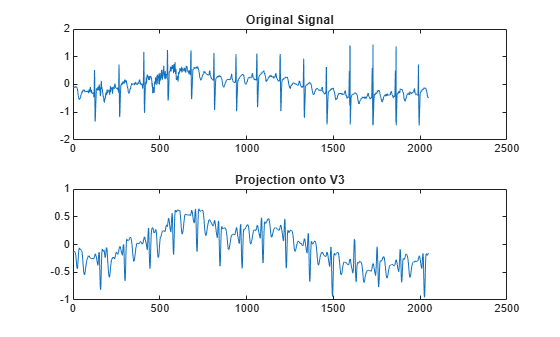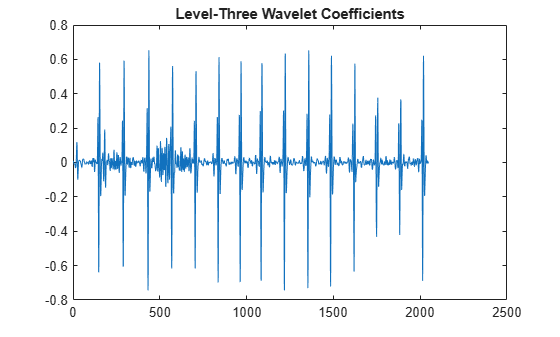Documentation

# imodwt

Inverse maximal overlap discrete wavelet transform

## Syntax

``xrec = imodwt(w)``
``xrec = imodwt(w,wname)``
``xrec = imodwt(w,Lo,Hi)``
``xrec = imodwt(___,lev)``
``xrec = imodwt(___,'reflection')``

## Description

example

````xrec = imodwt(w)` returns in `xrec` a reconstructed version of the signal. The reconstructed signal is based on `w`, the maximal overlap discrete wavelet transform (MODWT) coefficients and on the level of reconstruction, which defaults to zero. ```

example

````xrec = imodwt(w,wname)` reconstructs the signal using `wname`, the orthogonal wavelet. `wname` must be the same wavelet used to analyze the signal input to `modwt`. The reconstruction is up to level 0, which is a perfect reconstruction of the original signal.```

example

````xrec = imodwt(w,Lo,Hi)` reconstructs the signal using the orthogonal scaling filter `Lo` and the wavelet filter `Hi`. The `Lo` and `Hi` filters must be the same filters used to analyze the signal input to `modwt`. The reconstruction is up to level 0, which is a perfect reconstruction of the original signal.```

example

````xrec = imodwt(___,lev)` reconstructs the signal up to level `lev`. `xrec` is a projection onto the scaling space at level `lev`. ```

example

````xrec = imodwt(___,'reflection')` uses the reflection boundary condition in the reconstruction. If you specify `'reflection'`, `imodwt` assumes that the length of the original signal length is one half the number of columns in the input coefficient matrix. By default, ` imodwt` assumes periodic signal extension at the boundary.```

## Examples

collapse all

Obtain the MODWT of an ECG signal and demonstrate perfect reconstruction.

Load the ECG signal data and obtain the MODWT.

`load wecg;`

Obtain the MODWT and the Inverse MODWT.

```w = modwt(wecg); xrec = imodwt(w);```

Use the L-infinity norm to show that the difference between the original signal and the reconstruction is extremely small. The largest absolute difference between the original signal and the reconstruction is on the order of $1{0}^{-12}$, which demonstrates perfect reconstruction.

`norm(abs(xrec'-wecg),Inf)`
```ans = 2.3253e-12 ```

Obtain the MODWT of Deutsche Mark-U.S. Dollar exchange rate data and demonstrate perfect reconstruction.

Load the Deutsche Mark-U.S. Dollar exchange rate data.

`load DM_USD;`

Obtain the MODWT and the Inverse MODWT using the `'db2'` wavelet.

```wdm = modwt(DM_USD,'db2'); xrec = imodwt(wdm,'db2');```

Use the L-infinity norm to show that the difference between the original signal and the reconstruction is extremely small. The largest absolute difference between the original signal and the reconstruction is on the order of $1{0}^{-13}$, which demonstrates perfect reconstruction.

`norm(abs(xrec'-DM_USD),Inf)`
```ans = 1.6370e-13 ```

Obtain the MODWT of an ECG signal using the Fejer-Korovkin filters.

`load wecg;`

Create the 8-coefficient Fejer-Korovkin filters.

`[Lo,Hi] = wfilters('fk8');`

Obtain the MODWT and Inverse MODWT.

```wtecg = modwt(wecg,Lo,Hi); xrec = imodwt(wtecg,Lo,Hi);```

Plot the original data and the reconstruction.

```subplot(2,1,1) plot(wecg) title('ECG Signal'); subplot(2,1,2) plot(xrec) title('Reconstruction')```Obtain the MODWT of an ECG signal down to the maximum level and obtain the projection of the ECG signal onto the scaling space at level 3.

`load wecg;`

Obtain the MODWT.

`wtecg = modwt(wecg);`

Obtain the projection of the ECG signal onto ${V}_{3}$, the scaling space at level three by using the `imodwt` function.

`v3proj = imodwt(wtecg,3);`

Plot the original signal and the projection.

```subplot(2,1,1) plot(wecg) title('Original Signal') subplot(2,1,2) plot(v3proj) title('Projection onto V3')```Note that the spikes characteristic of the R waves in the ECG are missing in the ${V}_{3}$ approximation. You can see the missing details by examining the wavelet coefficients at level three.

Plot the level-three wavelet coefficients.

```figure plot(wtecg(3,:)) title('Level-Three Wavelet Coefficients')```Obtain the inverse MODWT using reflection boundary handling for Southern Oscillation Index data. The sampling period is one day. `imodwt` with the `'reflection'` option assumes that the input matrix, which is the `modwt` output, is twice the length of the original signal length. `imodwt` reflection boundary handling reduces the number of wavelet and scaling coefficients at each scale by half.

```load soi; wsoi = modwt(soi,4,'reflection'); xrecsoi = imodwt(wsoi,'reflection');```

Use the L-infinity norm to show that the difference between the original signal and the reconstruction is extremely small. The largest absolute difference between the original signal and the reconstruction is on the order of $1{0}^{-11}$, which demonstrates perfect reconstruction.

`norm(abs(xrecsoi'-soi),Inf)`
```ans = 1.6419e-11 ```

## Input Arguments

collapse all

MODWT transform, specified as a matrix of size L+1-by-N. `w` is the output of `modwt`, which is the MODWT of an N-point input signal down to level L. By default, `imodwt` assumes that you obtained the MODWT using the `'sym4'` wavelet with periodic boundary handling.

Data Types: `double`

Synthesis wavelet, specified as one of the following:

• `'haar'` — Haar wavelet

• `'dbN'` — Extremal phase Daubechies wavelet with `N` vanishing moments, where `N` is a positive integer from 1 to 45.

• `'symN'` — Symlets wavelet with `N` vanishing moments, where `N` is a positive integer from 2 to 45.

• `'coifN'` — Coiflets wavelet with `N` vanishing moments, where `N` is a positive integer from 1 to 5.

• `'fkN'` — Fejér-Korovkin wavelet with `N` coefficients, where `N = 4, 6, 8, 14, 18` and `22`.

The synthesis wavelet must be the same wavelet used in the analysis with `modwt`.

Scaling filter, specified as an even-length real-valued vector. You can specify `Lo` only if you do not specify `wname`. `Lo` must be the same scaling filter used in the analysis with `modwt`.

Wavelet filter, specified as an even-length real-valued vector. You can specify `Hi` only if you do not specify `wname`. `Hi` must be the same wavelet filter used in the analysis with `modwt`.

Reconstruction level, specified as a nonnegative integer between 0 and `size(w,1)-2`. The level must be less than the level used to obtain `w` from `modwt`. If `lev` is 0 and you do not modify the coefficients, `imodwt` produces a perfect reconstruction of the signal.

## Output Arguments

collapse all

Reconstructed version of the original signal based on the MODWT and the level of reconstruction, returned as a row vector.

 Percival, D. B., and A. T. Walden. Wavelet Methods for Time Series Analysis. Cambridge, UK: Cambridge University Press, 2000.

##### Support평가판 신청